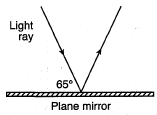Introduction to WavesSound Analysis LabReflection and Review

When a wave hits a boundary, three different events can occur. The wave may be:

• Reflected – wave bounces off a boundary
• Transmitted – wave is transmitted into the new medium
• Absorbed – energy of the wave is transferred into the boundary mediumThe law of reflection states that the angle at which a wave strikes a reflective medium (the angle of incidence, or) is equal to the angle at which a wave reflects off the medium (the angle of reflection, or). Put more simply,. In all cases, the angle of incidence and the angle of reflection are measured from a line perpendicular, or normal, to the reflecting surface.

Although all waves can exhibit these behaviors, electromagnetic light waves are typically considered for demonstration purposes. When a wave bounces off a reflective surface, the nature of its reflection depends largely on the nature of the surface. Rough surfaces tend to reflect light in a variety of directions in a process known as diffuse reflection. Diffuse reflection is the type of reflection typically observed off of pieces of paper. Smooth surfaces tend to reflect light waves in a more regular fashion, such that the reflected rays maintain parallel reflected rays. This process is known as specular reflection, and is commonly observed in mirrors.Question: The diagram represents a light ray reflecting from a plane mirror. Find the angle of reflection for the light ray.

Answer: The angle of reflection must be 25°, since the angle of incidence is 25° (note that angles are always measured from the normal!)

Review Problems

Key Points:

The velocity of a wave depends upon its medium.

The frequency of a wave determines its nature and remains constant from medium to medium.